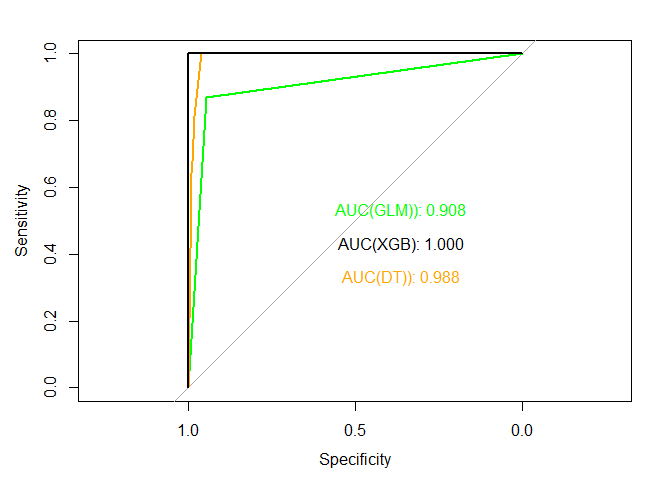## Libraries

Today we will work with the following libraries:

library(dplyr)
library(ggplot2)
library(ROSE) # Balancing
library(pROC) # AUC
library(reshape) #melt
library(stringr) # work with strings
library(rpart) # decision tree

library(xgboost) # for xgboost
library(caret) # for confusion matrix

Additional packages that may need to run the code:

# packages required to create a map
install.packages("maps")
install.packages("mapproj")

# package required printing important features for XGBoost
install.packages("Ckmeans.1d.dp")

# for confusion matrix
install.packages("e1071")

## Data preparation

df <- read.csv(file.choose()) # fraud.csv

Let’s explore the data:

str(df)
## 'data.frame':    108717 obs. of  11 variables:
##  $step : int 1 1 1 1 1 1 1 1 1 1 ... ##$ type          : Factor w/ 5 levels "CASH_IN","CASH_OUT",..: 4 4 5 2 4 4 4 4 4 3 ...
##  $amount : num 9840 1864 181 181 11668 ... ##$ nameOrig      : Factor w/ 108717 levels "C1000028246",..: 12889 37314 16984 99757 58719 103085 30706 51110 14751 92379 ...
##  $oldbalanceOrg : num 170136 21249 181 181 41554 ... ##$ newbalanceOrig: num  160296 19385 0 0 29886 ...
##  $nameDest : Factor w/ 55434 levels "C1000156006",..: 34340 35755 9983 8874 17883 45921 42245 47239 16753 6419 ... ##$ oldbalanceDest: num  0 0 0 21182 0 ...
##  $newbalanceDest: num 0 0 0 0 0 ... ##$ isFraud       : int  0 0 1 1 0 0 0 0 0 0 ...
##  $coord : Factor w/ 833 levels "","[-33.87144962, 151.20821275]",..: 97 663 765 34 87 709 129 565 272 458 ... We have next features: • step - maps a unit of time in the real world. In this case 1 step is 1 hour of time. • type - type of transaction that was executed. • amount - amount of the transaction in local currency • nameOrig - customer who started the transaction. • oldbalanceOrg - initial balance before the transaction. • newbalanceOrig - customer’s balance after the transaction. • nameDest - recipient ID of the transaction. • oldbalanceDest - initial recipient balance before the transaction. • newbalanceDest - recipient’s balance after the transaction. • isFraud - identifies a fraudulent transaction (1) and non fraudulent (0). ## Data exploration Let’s explore our data by looking at how much fraud attempts there were for each type of transaction. df %>% group_by(type) %>% summarise(isFraud = sum(isFraud)) ## # A tibble: 5 x 2 ## type isFraud ## <fct> <int> ## 1 CASH_IN 0 ## 2 CASH_OUT 61 ## 3 DEBIT 0 ## 4 PAYMENT 0 ## 5 TRANSFER 59 Based on result we can conclude that there aren’t many atempts in fraud. Now let’s try to find amount of money that could be lost due to the fraud. First, let’s calculate total amount of cash: df %>% group_by(type) %>% summarise(amount = sum(amount)) ## # A tibble: 5 x 2 ## type amount ## <fct> <dbl> ## 1 CASH_IN 3846102487. ## 2 CASH_OUT 6615077732. ## 3 DEBIT 4375667. ## 4 PAYMENT 466499907. ## 5 TRANSFER 8170484901. Now let’s calculate the percentage of money that could be lost: df %>% group_by(type) %>% mutate(totAmount = sum(amount)) %>% filter(isFraud > 0) %>% summarise(amount = sum(amount), percent = amount / last(totAmount)) ## # A tibble: 2 x 3 ## type amount percent ## <fct> <dbl> <dbl> ## 1 CASH_OUT 33551538. 0.00507 ## 2 TRANSFER 34615462. 0.00424 #Plotting coordinates on geomap Now, let’s try to plot locations on the map, where transactions were executed. Let’s look at coordinates: head(df$coord)
##  [29.98384194, -95.33664018] [40.80718573, -73.95477259]
##  [42.3658858, -71.01423374]  [24.55401318, -81.80300774]
##  [29.7420124, -95.5606921]   [41.86591215, -87.6231126]
## 833 Levels:  [-33.87144962, 151.20821275] ... [59.38247253, 18.00789007]

There are some empty values in coord column:

data_with_coord <- df[df$coord != "",] Next, to plot coordinates on the map, we have to separate them. tmp <- data.frame(str_split_fixed(data_with_coord$coord, ", ", 2))
tmp$X1 <- gsub('^.', '', tmp$X1) # Remove [
tmp$X2 <- gsub('.$', '', tmp$X2) # Remove ] colnames(tmp) <- c("lat", "lon") # Set colnames data_with_coord$lat <- as.numeric(tmp$lat) # Add latitude column to airline_data data_with_coord$lon <- as.numeric(tmp$lon) # Add longtitude column to airline_data Now let’s plot the map: # Setting world data WorldData <- map_data('world') WorldData <- fortify(WorldData) # Setting world map geomap <- ggplot() + geom_map( data=WorldData, map=WorldData, aes(x=long, y=lat, group=group, map_id=region), fill="white", colour="#7f7f7f", size=0.5) # Setting map borders geomap <- geomap + coord_map("rectangular", lat0=0, xlim=c(-180,180), ylim=c(-60, 90)) # Setting coordinates geomap <- geomap + geom_point( aes(x = lon, y = lat, color = type), alpha = 1, data = data_with_coord, size = 2) # Setting colors geomap <- geomap + scale_colour_manual(values=c("orange", "purple", "red", "darkgreen", "blue")) geomap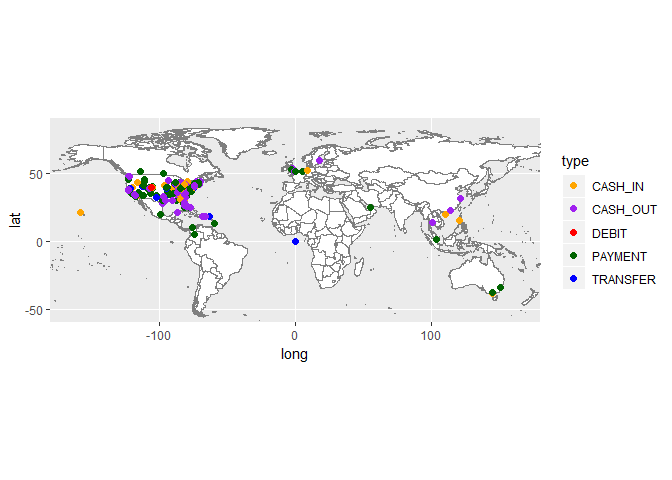df$coord <- NULL

# Prediction

## Data preparation

Let’s look at our data once more:

str(df)
## 'data.frame':    108717 obs. of  10 variables:
##  $step : int 1 1 1 1 1 1 1 1 1 1 ... ##$ type          : Factor w/ 5 levels "CASH_IN","CASH_OUT",..: 4 4 5 2 4 4 4 4 4 3 ...
##  $amount : num 9840 1864 181 181 11668 ... ##$ nameOrig      : Factor w/ 108717 levels "C1000028246",..: 12889 37314 16984 99757 58719 103085 30706 51110 14751 92379 ...
##  $oldbalanceOrg : num 170136 21249 181 181 41554 ... ##$ newbalanceOrig: num  160296 19385 0 0 29886 ...
##  $nameDest : Factor w/ 55434 levels "C1000156006",..: 34340 35755 9983 8874 17883 45921 42245 47239 16753 6419 ... ##$ oldbalanceDest: num  0 0 0 21182 0 ...
##  $newbalanceDest: num 0 0 0 0 0 ... ##$ isFraud       : int  0 0 1 1 0 0 0 0 0 0 ...

## Balancing data

First issue that we have to address is data balancing. Currently we have only few observations with the fraud, so let’s perform bothsampling and check the result.

df_b <- ovun.sample(isFraud~. , data = df, method = "both",
p=0.5, seed = 1000)$data ggplot(df_b, aes(x = isFraud, fill = as.factor(isFraud))) + geom_bar()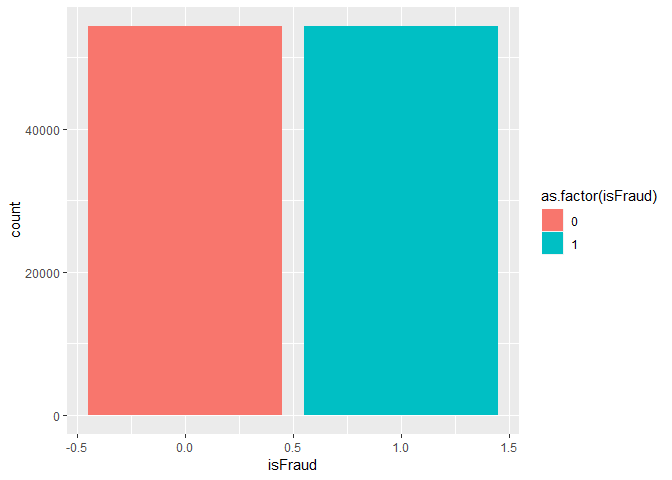### Data preparation To use XGBoost & Logistic Regression we need to convert features to numerics and scale them. df_b$type <- as.numeric(df_b$type) df_b$nameOrig <- as.numeric(df_b$nameOrig) df_b$nameDest <- as.numeric(df_b$nameDest) Next, we have to rescale the features: scaled_df <- df_b %>% mutate(step = scale(step), type = scale(type), amount = scale(amount), nameOrig = scale(nameOrig), oldbalanceOrg = scale(oldbalanceOrg), newbalanceOrig = scale(newbalanceOrig), nameDest = scale(nameDest), oldbalanceDest = scale(oldbalanceDest), newbalanceDest = scale(newbalanceDest) ) ## Logistic regression Let’s try logistic regression on our data: Splitting the data: samples <- sample.int(n = nrow(scaled_df), size = floor(0.8*nrow(scaled_df)), replace = F) train <- scaled_df[samples,] test <- scaled_df[-samples,] Now, let’s check how logistic regression performs on our data. First, we train the model: glm <- glm(isFraud ~ ., train, family=binomial) And finaly evaluate the result: pred_glm_scores <- predict(glm, test) threshold <- 0.5 # We set threshold for obtained scores glm_predict_boolean <- ifelse(pred_glm_scores >= threshold,1,0) glm_cm <- confusionMatrix(as.factor(glm_predict_boolean), as.factor(test$isFraud))
glm_cm
## Confusion Matrix and Statistics
##
##           Reference
## Prediction     0     1
##          0 10427  1391
##          1   599  9327
##
##                Accuracy : 0.9085
##                  95% CI : (0.9046, 0.9123)
##     No Information Rate : 0.5071
##     P-Value [Acc > NIR] : < 2.2e-16
##
##                   Kappa : 0.8167
##
##  Mcnemar's Test P-Value : < 2.2e-16
##
##             Sensitivity : 0.9457
##             Specificity : 0.8702
##          Pos Pred Value : 0.8823
##          Neg Pred Value : 0.9397
##              Prevalence : 0.5071
##          Detection Rate : 0.4795
##    Detection Prevalence : 0.5435
##       Balanced Accuracy : 0.9079
##
##        'Positive' Class : 0
## 
print(paste("Recall of Logistic Regression is:", glm_cm$byClass["Recall"])) ##  "Recall of Logistic Regression is: 0.945673861781244" print(paste("Precision of Logistic Regression is:", glm_cm$byClass["Precision"]))
##  "Precision of Logistic Regression is: 0.882298189202911"
print(paste("F1 of Logistic Regression is:", glm_cm$byClass["F1"])) ##  "F1 of Logistic Regression is: 0.9128874102609" We can also create ROC and find AUC roc_glm <- roc(test$isFraud, glm_predict_boolean)
plot.roc(roc_glm, col="orange")
text(0.36, 0.53, labels=sprintf("AUC(GLM)): %0.3f", auc(roc_glm)), col="orange")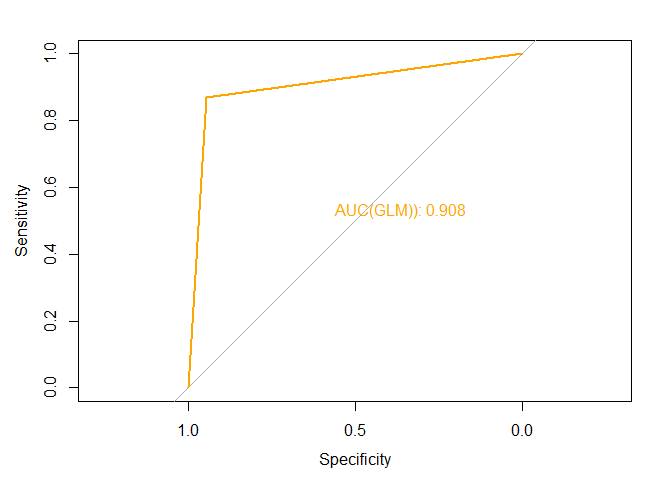## Decision Tree

Let’s try decison tree on our data and see the result.

tree <- rpart(isFraud~., train)

And finaly evaluate the result:

pred_tree_scores <- predict(tree, test)

threshold <-  0.5 # We set threshold for obtained scores
tree_predict_boolean <- ifelse(pred_tree_scores >= threshold,1,0)

tree_cm <- confusionMatrix(as.factor(tree_predict_boolean), as.factor(test$isFraud)) tree_cm ## Confusion Matrix and Statistics ## ## Reference ## Prediction 0 1 ## 0 10579 0 ## 1 447 10718 ## ## Accuracy : 0.9794 ## 95% CI : (0.9775, 0.9813) ## No Information Rate : 0.5071 ## P-Value [Acc > NIR] : < 2.2e-16 ## ## Kappa : 0.9589 ## ## Mcnemar's Test P-Value : < 2.2e-16 ## ## Sensitivity : 0.9595 ## Specificity : 1.0000 ## Pos Pred Value : 1.0000 ## Neg Pred Value : 0.9600 ## Prevalence : 0.5071 ## Detection Rate : 0.4865 ## Detection Prevalence : 0.4865 ## Balanced Accuracy : 0.9797 ## ## 'Positive' Class : 0 ##  print(paste("Recall of Decision Tree is:", tree_cm$byClass["Recall"]))
##  "Recall of Decision Tree is: 0.959459459459459"
print(paste("Precision of Decision Tree is:", tree_cm$byClass["Precision"])) ##  "Precision of Decision Tree is: 1" print(paste("F1 of Decision Tree is:", tree_cm$byClass["F1"]))
##  "F1 of Decision Tree is: 0.979310344827586"

We can also create ROC and find AUC

matrix_test <- xgb.DMatrix(as.matrix(test %>% select(-isFraud)), label = test$isFraud) ### Run the model with XGBoost watchlist <- list(train = matrix_train, cv = matrix_test) params <- list( "objective" = "binary:logitraw", #Specify the learning task and the corresponding learning objective. "eval_metric" = "auc" ) model_xgb <- xgb.train(params=params, data=matrix_train, maximize=TRUE, # larger the evaluation score the better nrounds=50, # max number of boosting iterations nthread=3, # number of threads early_stopping_round=10, # stop if the performance doesn't improve for 10 rounds watchlist = watchlist, # named list of xgb.DMatrix datasets to use for evaluating model performance print_every_n=5) # prints metrics for every 5th iteration ##  train-auc:0.994021 cv-auc:0.993619 ## Multiple eval metrics are present. Will use cv_auc for early stopping. ## Will train until cv_auc hasn't improved in 10 rounds. ## ##  train-auc:0.998888 cv-auc:0.998773 ##  train-auc:0.999747 cv-auc:0.999532 ##  train-auc:0.999971 cv-auc:0.999984 ##  train-auc:0.999992 cv-auc:0.999999 ##  train-auc:0.999996 cv-auc:0.999999 ##  train-auc:0.999998 cv-auc:1.000000 ## Stopping. Best iteration: ##  train-auc:0.999992 cv-auc:1.000000 ### Variable importance importance <- xgb.importance(colnames(matrix_train), model = model_xgb) xgb.ggplot.importance(importance)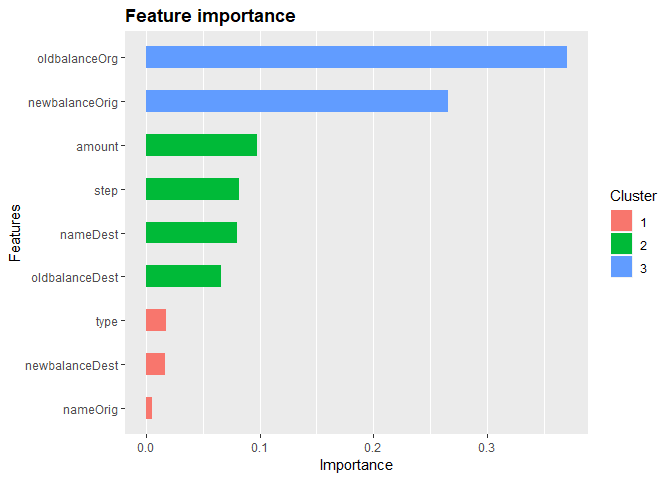### Ploting AUC Let’s see how AUC changes with next iterations of model training melted <- melt(model_xgb$evaluation_log, id.vars="iter")
ggplot(data=melted, aes(x=iter, y=value, group=variable, color = variable)) + geom_line()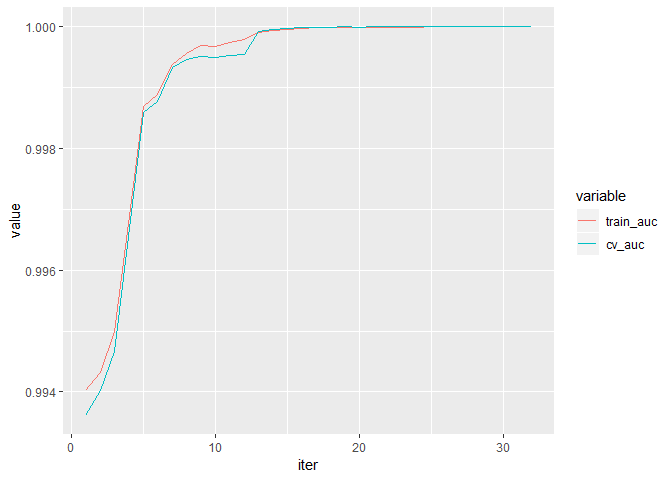### Prediction

Now let’s predict fraud using test data:

xgb_predict_scores <- predict(model_xgb, matrix_test)
threshold <-  0.5 # We set threshold for obtained scores
xgb_predict_boolean <- ifelse(xgb_predict_scores >= threshold,1,0)

And evaluate the result by creating confusion matrix:

xgb_cm <- confusionMatrix(as.factor(xgb_predict_boolean), as.factor(test$isFraud)) xgb_cm ## Confusion Matrix and Statistics ## ## Reference ## Prediction 0 1 ## 0 10916 0 ## 1 16 10812 ## ## Accuracy : 0.9993 ## 95% CI : (0.9988, 0.9996) ## No Information Rate : 0.5028 ## P-Value [Acc > NIR] : < 2.2e-16 ## ## Kappa : 0.9985 ## ## Mcnemar's Test P-Value : 0.0001768 ## ## Sensitivity : 0.9985 ## Specificity : 1.0000 ## Pos Pred Value : 1.0000 ## Neg Pred Value : 0.9985 ## Prevalence : 0.5028 ## Detection Rate : 0.5020 ## Detection Prevalence : 0.5020 ## Balanced Accuracy : 0.9993 ## ## 'Positive' Class : 0 ##  We can access different metrics from our confusion matrix. print(paste("Recall of XGBoost is:", xgb_cm$byClass["Recall"]))
##  "Recall of XGBoost is: 0.998536406878888"
print(paste("Precision of XGBoost is:", xgb_cm$byClass["Precision"])) ##  "Precision of XGBoost is: 1" print(paste("F1 of XGBoost is:", xgb_cm$byClass["F1"]))
##  "F1 of XGBoost is: 0.999267667521055"

We can also create ROC and find AUC

roc_xgb <- roc(test\$isFraud, predict(model_xgb, matrix_test, type = "prob"))
plot.roc(roc_xgb)
text(0.36, 0.53, labels=sprintf("AUC(XGB)): %0.3f", auc(roc_xgb)), col="black")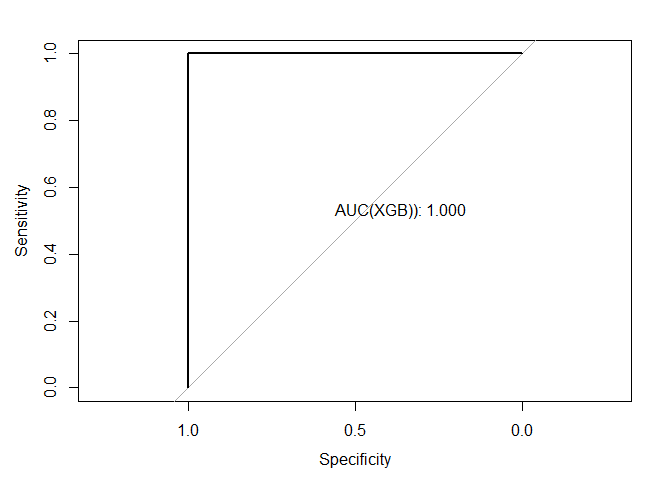## Result

Let’s compare all 3 AUC’s and select the best one.

plot.roc(roc_glm, col="green")
text(0.36, 0.53, labels=sprintf("AUC(GLM)): %0.3f", auc(roc_glm)), col="green")

lines(roc_dt, col="orange")
text(0.36, 0.33, labels=sprintf("AUC(DT)): %0.3f", auc(roc_dt)), col="orange")

lines(roc_xgb, col="black")
text(0.36, 0.43, labels=sprintf("AUC(XGB): %0.3f", auc(roc_xgb)), col="black")